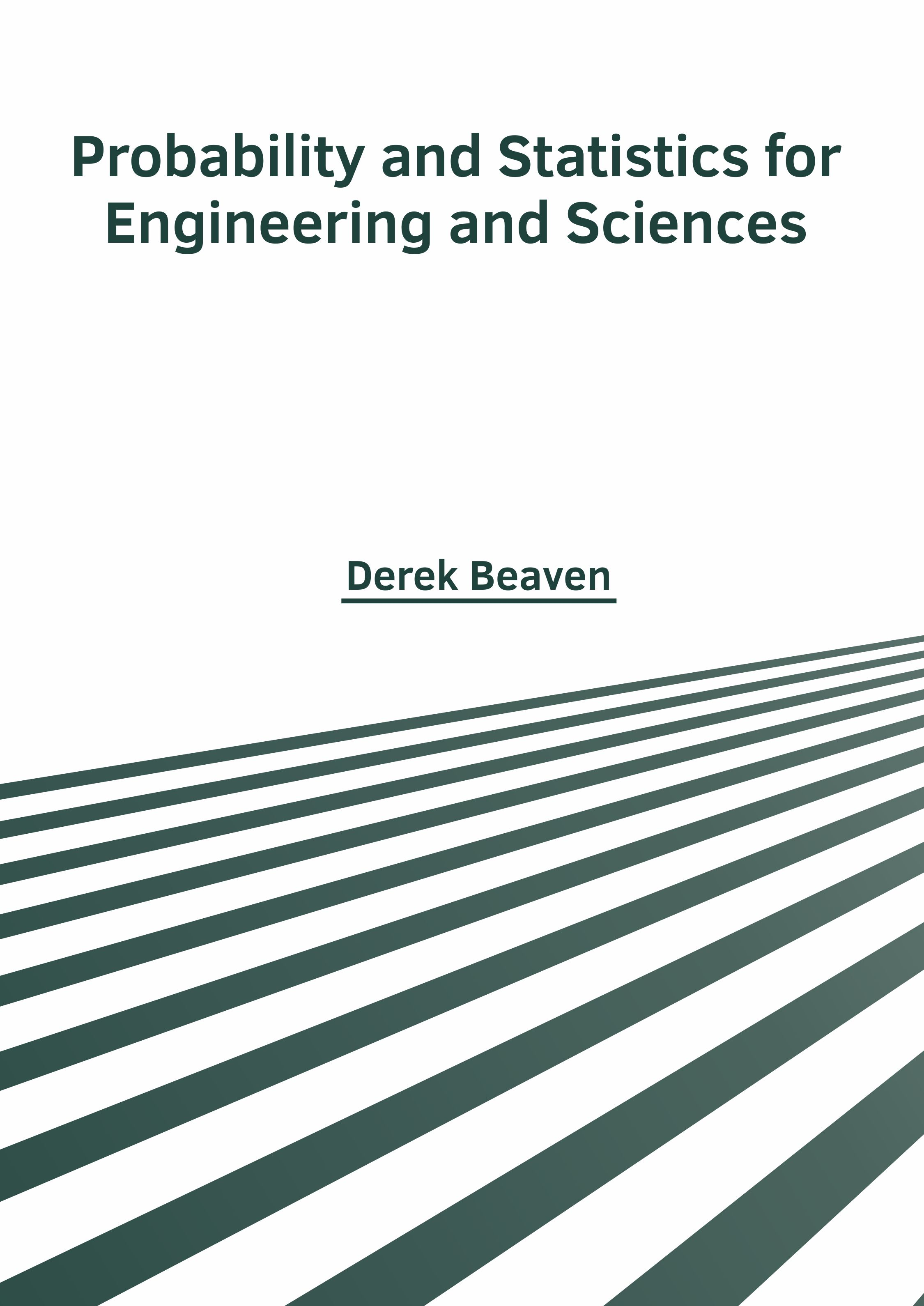BROWSE BY

#### Author

Results 1 - 2 of 2Probability and Statistics for Engineering and Sciences Author : Derek Beaven Subject : Mathematics ISBN :9781632387349 Probability and Statistics are two closely related sub-disciplines of mathematical. Statistics is a mathematical branch that deals with data collection, organization, interpretation, presentation and Read MoreProbability and Statistics: Theories and Applied Principles Author : Derek Beaven Subject : Mathematics ISBN :9781632385802 The subjects of statistics and probability are interrelated and are generally studied together. They can be applied in a number of fields such as finance, machine learning, game theory, etc. The book presents Read More
Results 1 - 2 of 2